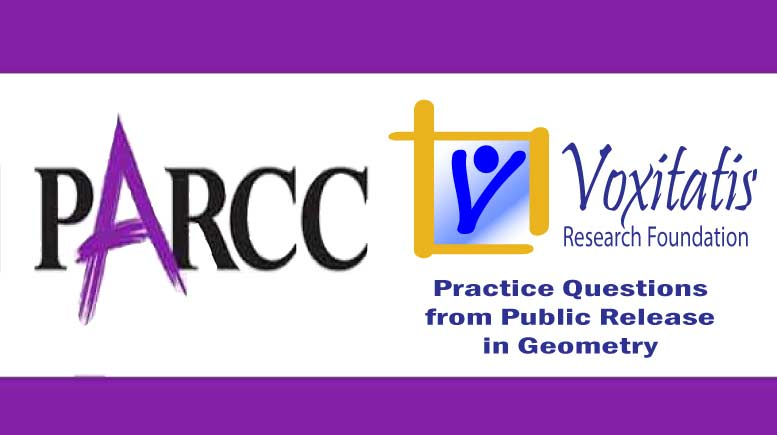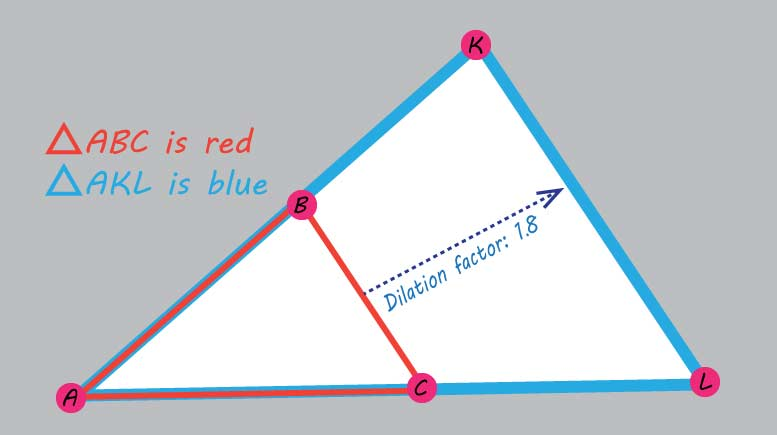Wednesday, October 28, 2020# Geometry PARCC question: triangle dilation

-

#### The following multiple-select question, explained here in hopes of helping geometry students in Maryland and Illinois prepare for the PARCC test near the end of this school year, appears on the released version of PARCC’s Spring 2015 test in geometry, here:ΔABC is dilated from center A by a factor not equal to 1 to form ΔAKL. Which of the statements must be true?

Select all that apply.

1.$\overline{AB}\textrm{ and }\overline{AK}\textrm{ lie on the same line.}$
2.$\textrm{The line containing }\overline{BC}\textrm{ is parallel to the line containing }\overline{KL}\textrm{.}$
3.$\angle\textrm{ABC} \cong\angle\textrm{ACB}$
4.$\angle\textrm{ABC} \cong\angle\textrm{AKL}$
5.$\Delta\textrm{ABC} \sim\Delta\textrm{AKL}$
6.$\Delta\textrm{ABC} \cong\Delta\textrm{AKL}$

Correct answers: 1, 2, 4, and 5 all must be true.

PARCC evidence statement(s) tested: G-SRT.2:

Given two figures, use the definition of similarity in terms of similarity transformations to decide if they are similar.

The “explain” part of standard G-SRT.2 is not assessed here.

The evidence statement above references Math Practice 7 in the Common Core: Look for and make use of structure. Mathematically proficient students look closely to discern a pattern or structure. … Students will see 7 × 8 equals the well remembered 7 × 5 + 7 × 3, in preparation for learning about the distributive property. In the expression x2 + 9x + 14, older students can see the 14 as 2 × 7 and the 9 as 2 + 7. They recognize the significance of an existing line in a geometric figure and can use the strategy of drawing an auxiliary line for solving problems. They also can step back for an overview and shift perspective. They can see complicated things, such as some algebraic expressions, as single objects or as being composed of several objects. For example, they can see 5 – 3(x – y)2 as 5 minus a positive number times a square and use that to realize that its value cannot be more than 5 for any real numbers x and y.

The question tests students’ understanding of the high school Common Core geometry standard HSG.SRT.A, found under High School: Geometry, Similarity, Right Triangles, & Trigonometry (understand similarity in terms of similarity transformations), which states that they should be able to “verify experimentally the properties of dilations given by a center and a scale factor,” including an understanding that [A.1.A] “a dilation takes a line not passing through the center of the dilation to a parallel line and leaves a line passing through the center unchanged” and [A.1.B] “the dilation of a line segment is longer or shorter in the ratio given by the scale factor.” Students can, [A.2] “given two figures, use the definition of similarity in terms of similarity transformations to decide if they are similar …”; and [A.3] “use the properties of similarity transformations to establish the AA criterion for two triangles to be similar.”Option 1 is correct. Any dilation leaves a line passing through the center unchanged. Since the center of the dilation is at A, any line going through that center will be the same line before and after the dilation.

Option 2 is correct. A dilation will take any line not passing through the center of the dilation, in this case the line with segment BC on it, to a parallel line, which, in this case, is the line with segment KL on it.

Option 3 is incorrect. The problem provided no information that angle ABC was congruent to angle ACB. That would mean this was an isosceles triangle, which I suppose it could be, but you weren’t given that information. Don’t assume something so blatant on a test. If the test writers wanted ΔABC to be isosceles, they would have told you.

Option 4 is correct. The two triangles have every corresponding angle congruent. Here’s a rule of thumb, which could come in handy when you’re doing a geometric proof: Dilations of entire figures leave all angles unchanged. It’s a property of dilations.

Option 5 is correct. Following from option (4) above, if all the angles in ΔABC are unchanged after the transformation to ΔAKL, then by the AA (angle-angle) similarity theorem, the two triangles are similar to each other.

Option 6 is incorrect. The two triangles aren’t congruent to each other. A dilation by a factor other than 1 changes the size of the original figure, and congruent triangles have the same shape and size. Shape, yes, but not size in this case.

## Resources for further study

Math Is Fun, maintained by Rod Pierce, has a nice page that explains the difference between similar and congruent figures. This is elementary stuff, but just as a refresher, check it out, and think about how it applies to doing dilations.

The Khan Academy, developed by Sal Khan, an engineer who has created a library of thousands of video lessons, has more than 20 lesson videos about transforming geometric figures, including expanding and shrinking them by dilating from a certain point. The landing page for the lessons is here.

Chapter 8, Section 8.2, of the book Geometry for Enjoyment and Challenge by Richard Rhoad et al, all teachers from Illinois, deals with similarity in polygons. Similar polygons, they write, are those in which

1. The ratios of the measures of corresponding sides are equal
2. Corresponding angles are congruent

(Richard Rhoad, George Milauskas, and Robert Whipple. Geometry for Enjoyment and Challenge, new edition. Evanston, Ill.: McDougal Littell, a division of Houghton Mifflin Company, 1991. The book is used in several geometry classes taught in Illinois high schools.)

## Analysis of this question and online accessibility

The question measures knowledge of the Common Core standard it purports to measure and tests students’ ability apply the principles of dilations to work with triangle similarity theorems. It is considered to have a low cognitive demand.

The question can be tested online and should yield results that are as valid and reliable as those obtained on paper.

No special accommodation challenges can be identified with this question, so the question is considered fair.

## Challenge

A building casts a 98-foot shadow at the same time that a 29-foot flagpole casts as 31.5-foot shadow. About how tall is the building?

## Purpose of this series of posts

Voxitatis is developing blog posts that address every algebra 1 question released to the public by the Partnership for Assessment of Readiness for College and Careers, or PARCC, in order to help students prepare to take the test this spring.

Our total release will run from February 27 through March 15, with one or two questions discussed per day. Then we’ll move to geometry at the end of March, algebra 2 during the first half of April, and eighth grade during the last half of April.Paul Katulahttp://news.schoolsdo.org
Paul Katula is the executive editor of the Voxitatis Research Foundation, which publishes this blog. For more information, see the About page.

### Volleyball teams continue to dig for a cure

0
Girls' volleyball teams, whether in clubs or in high schools, have a common cause in October: doing what they can to cure breast cancer.